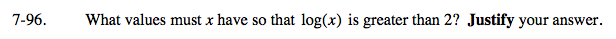Home > A2C > Chapter 7 > Lesson 7.2.1 > Problem7-96

7-96.

What values must x have so that log(x) is greater than 2? Justify your answer. Homework Help ✎Solve the equation log(x) = 2, and then test values to find where log(x) > 2.

Remember log(x) means log10(x). Rewrite the equation in exponential form.